1│2│3│4四元數與Cayley數 （第 3 頁） 余文卿 ．原載於數學傳播十五卷二期 ．作者當時任教於中正大學數學所 •對外搜尋關鍵字

 三、Cayley數

Cayley 數是由四元數 H 加入另一單位 e 而得，即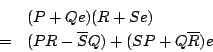Cayley 數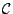中，除 H 中原來就有的 4 個單位 1,i,j,k 外，另由 e 與這四個單位相乘而得出四個單位。因此 C 中共有 8 個單位； 而以 e0(=1),e1,e2,e3,e4,e5,e6,e7 分別表示，這些單位與原先單位 i,j,k,e 的關係為

e0=1,e1=j,e2=i,e3=e,e4=-k,e5=ie,e6=ke,e7=je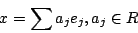1.2.3. e1e2e4=e2e3e5=e3e4e6=e4e5e7=e5e6e1=e6e7e2=e7e1e3=-e0

(xy)z=x(yz)

1. e1(e2e4)=(e1e2)e4=-e0
2. e1e2=-e2e1=e4,e2e4=-e4e2=e2,e4e1=-e1e4=e2

(e1e2)e3=e4e3=-e3e4=-e6

e1(e2e3)=e1e5=e6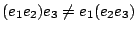Cayley數中，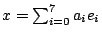的共軛數定為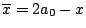，亦即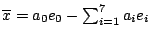。又範數N的定義是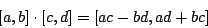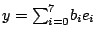代入 N(XY)=N(X)N(Y)中即得出下 面的等式：（若有指正、疑問……，可以在此 留言 或 寫信 給我們。）EpisteMath (c) 2000 中央研究院數學所、台大數學系 各網頁文章內容之著作權為原著作人所有
 編輯：許明吉 ／ 校對：黃怡碧 最後修改日期：4/26/2002